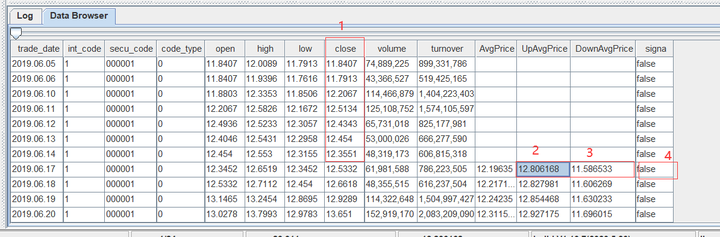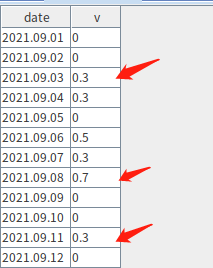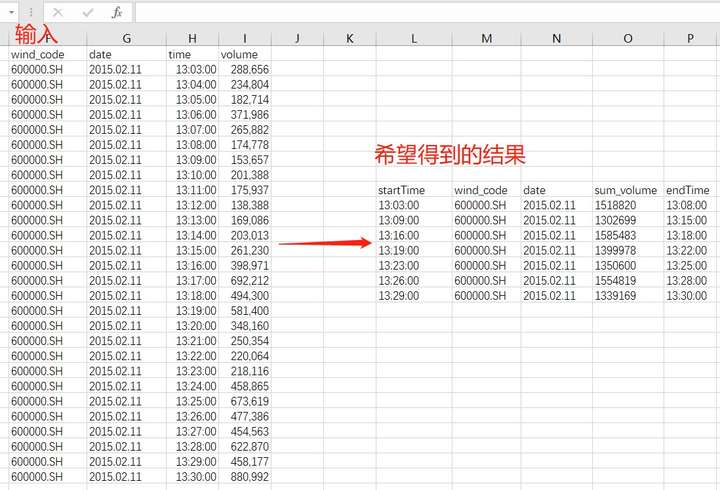# DolphinDB 函数化编程案例教程

DolphinDB支持函数化编程：函数对象可以作为高阶函数的参数。这提高了代码表达能力，可以简化代码，复杂的任务可以通过一行或几行代码完成。

1. 数据导入
2. Lambda表达式
3. 高阶函数使用案例
4. 部分应用案例
5. 金融场景相关案例
6. 机器学习相关案例

## 1. 数据导入

### 1.1 整型时间转化为 TIME 格式并导入

CSV 数据文件中常用整数表示时间，如 “93100000” 表示 “9:31:00.000”。为了便于查询分析，建议将这类数据转换为时间类型，再存储到 DolphinDB 数据库中。

``````symbol,exchange,cycle,tradingDay,date,time,open,high,low,close,volume,turnover,unixTime
000001,SZSE,1,20180102,20180102,93100000,13.35,13.39,13.35,13.38,2003635,26785576.72,1514856660000
000001,SZSE,1,20180102,20180102,93200000,13.37,13.38,13.33,13.33,867181
......``````

（1）建库

``````login(`admin,`123456)
dataFilePath="/home/data/candle_201801.csv"
dbPath="dfs://DolphinDBdatabase"
db=database(dbPath,VALUE,2018.01.02..2018.01.30)``````

（2）建表

``````schemaTB=extractTextSchema(dataFilePath)
update schemaTB set type="TIME" where name="time"
tb=table(1:0, schemaTB.name, schemaTB.type)
tb=db.createPartitionedTable(tb, `tb1, `date);``````

（3）导入数据

``````def i2t(mutable t){
return t.replaceColumn!(`time, t.time.format("000000000").temporalParse("HHmmssSSS"))
}``````

``tmpTB=loadTextEx(dbHandle=db, tableName=`tb1, partitionColumns=`date, filename=dataFilePath, transform=i2t);``

（4）查询数据

``````select top 2 * from loadTable(dbPath,`tb1);

symbol exchange cycle tradingDay date       time               open  high  low   close volume  turnover   unixTime
------ -------- ----- ---------- ---------- --------------     ----- ----- ----- ----- ------- ---------- -------------
000001 SZSE     1     2018.01.02 2018.01.02 09:31:00.000       13.35 13.39 13.35 13.38 2003635 2.678558E7 1514856660000
000001 SZSE     1     2018.01.02 2018.01.02 09:32:00.000       13.37 13.38 13.33 13.33 867181  1.158757E7 1514856720000``````

``````login(`admin,`123456)
dataFilePath="/home/data/candle_201801.csv"
dbPath="dfs://DolphinDBdatabase"
db=database(dbPath,VALUE,2018.01.02..2018.01.30)
schemaTB=extractTextSchema(dataFilePath)
update schemaTB set type="TIME" where name="time"
tb=table(1:0,schemaTB.name,schemaTB.type)
tb=db.createPartitionedTable(tb,`tb1,`date);

def i2t(mutable t){
return t.replaceColumn!(`time,t.time.format("000000000").temporalParse("HHmmssSSS"))
}

### 1.2 有纳秒时间戳的文本导入

``````SendingTimeInNano#securityID#origSendingTimeInNano#bidSize
1579510735948574000#27522#1575277200049000000#1
1579510735948606000#27522#1575277200049000000#2
...``````

（1）建库建表

``````dbSendingTimeInNano = database(, VALUE, 2020.01.20..2020.02.22);
dbSecurityIDRange = database(, RANGE,  0..10001);
db = database("dfs://testdb", COMPO, [dbSendingTimeInNano, dbSecurityIDRange]);

nameCol = `SendingTimeInNano`securityID`origSendingTimeInNano`bidSize;
typeCol = [`NANOTIMESTAMP,`INT,`NANOTIMESTAMP,`INT];
schemaTb = table(1:0,nameCol,typeCol);

db = database("dfs://testdb");
nx = db.createPartitionedTable(schemaTb, `nx, `SendingTimeInNano`securityID);``````

（2）导入数据

``````def dataTransform(mutable t){
return t.replaceColumn!(`SendingTimeInNano, nanotimestamp(t.SendingTimeInNano)).replaceColumn!(`origSendingTimeInNano, nanotimestamp(t.origSendingTimeInNano))
}``````

``````dbSendingTimeInNano = database(, VALUE, 2020.01.20..2020.02.22);
dbSecurityIDRange = database(, RANGE,  0..10001);
db = database("dfs://testdb", COMPO, [dbSendingTimeInNano, dbSecurityIDRange]);

nameCol = `SendingTimeInNano`securityID`origSendingTimeInNano`bidSize;
typeCol = [`NANOTIMESTAMP,`INT,`NANOTIMESTAMP,`INT];
schemaTb = table(1:0,nameCol,typeCol);

db = database("dfs://testdb");
nx = db.createPartitionedTable(schemaTb, `nx, `SendingTimeInNano`securityID);

def dataTransform(mutable t){
return t.replaceColumn!(`SendingTimeInNano, nanotimestamp(t.SendingTimeInNano)).replaceColumn!(`origSendingTimeInNano, nanotimestamp(t.origSendingTimeInNano))
}

## 2. Lambda 表达式

DolphinDB 中可以创建自定义函数，可以是命名函数或者匿名函数（通常为 lambda 表达式）。

``````x = 1..10
each(x -> pow(x,2), x)``````

## 3. 高阶函数使用案例

### 3.1.1 将两个向量或矩阵，两两组合作为参数来调用函数

`cross` 函数的伪代码如下：

``````for(i:0~(size(X)-1)){
for(j:0~(size(Y)-1)){
result[i,j]=<function>(X[i], Y[j]);
}
}
return result;``````

``````def matlab_cov(mutable matt){
nullFill!(matt,0.0)
rowss,colss=matt.shape()
msize = min(rowss, colss)
df=matrix(float,msize,msize)
for (r in 0..(msize-1)){
for (c in 0..(msize-1)){
df[r,c]=covar(matt[:,r],matt[:,c])
}
}
return df
}``````

``cross(covar, matt)``

### 3.1.2 计算股票两两之间的相关性

``````login("admin","123456")
dbPath="dfs://tushare"
yearRange=date(2008.01M + 12*0..22)
if(existsDatabase(dbPath)){
dropDatabase(dbPath)
}
type1=`SYMBOL`NANOTIMESTAMP`DOUBLE`DOUBLE`DOUBLE`DOUBLE`DOUBLE`DOUBLE`DOUBLE`DOUBLE`DOUBLE
db=database(dbPath,RANGE,yearRange)

``retMatrix=exec pct_change/100 as ret from daily_line pivot by trade_date, ts_code``

`exec`  `pivot by` 是 DolphinDB 编程语言的特点之一。`exec`  `select` 的用法相同，但 `select` 语句仅可生成表, `exec` 语句可以生成向量。`pivot by` 用于重整维度，与 `exec` 一起使用时会生成一个矩阵。

``corrMatrix=cross(corr,retMatrix)``

``````syms=(exec count(*) from daily_line group by ts_code).ts_code
syms="C"+strReplace(syms, ".", "_")
mostCorrelated=select * from table(corrMatrix.columnNames() as ts_code, corrMatrix).rename!([`ts_code].append!(syms)).unpivot(`ts_code, syms).rename!(`ts_code`corr_ts_code`corr) context by ts_code having rank(corr,false) between 1:10``````

``select * from table(corrMatrix.columnNames() as ts_code, corrMatrix).rename!([`ts_code].append!(syms)).unpivot(`ts_code, syms)``

``````ts_code   valueType  value
--------- ---------- -----------------
000001.SZ C600539_SH 1
000002.SZ C600539_SH 0.581235290880416
000004.SZ C600539_SH 0.277978963095669
000005.SZ C600539_SH 0.352580116619933
000006.SZ C600539_SH 0.5056164472398
......``````

``````login("admin","123456")

retMatrix=exec pct_change/100 as ret from daily_line pivot by trade_date,ts_code
corrMatrix=cross(corr,retMatrix)

syms=(exec count(*) from daily_line group by ts_code).ts_code
syms="C"+strReplace(syms, ".", "_")
mostCorrelated=select * from table(corrMatrix.columnNames() as ts_code, corrMatrix).rename!([`ts_code].append!(syms)).unpivot(`ts_code, syms).rename!(`ts_code`corr_ts_code`corr) context by ts_code having rank(corr,false) between 1:10``````

### 3.2.1 获取数据表各个列的 NULL 值个数

``each(x->x.size() - x.count(), t.values())``

### 3.2.2 去除表中存在 NULL 值的行

``````sym = take(`a`b`c, 110)
id = 1..100 join take(int(),10)
id2 =  take(int(),10) join 1..100
t = table(sym, id,id2)``````

``t[each(x -> !(x.id == NULL || x.id2 == NULL), t)]``

``t[each(x -> all(isValid(x.values())), t)]``

DolphinDB 采用列式存储，列操作较行操作具有更佳的性能。我们可以调用高阶函数 `each` 对表的每一列分别应用 `isValid` 函数，返回一个结果矩阵。通过 `rowAnd` 判断矩阵的每一行是否存在 0 值。

``t[each(isValid, t.values()).rowAnd()]``

``The number of cells in a matrix can't exceed 2 billions.``

``t[reduce(def(x,y) -> x and isValid(y), t.values(), true)]``

### 3.2.3 按行处理与按列处理性能比较案例

``t=table(take("aaaa_bbbb", 1000000) as str);``

``each(x -> split(x, '_').reverse().concat('_'), t[`str])``

``````pos = strpos(t[`str], "_")
substr(t[`str], pos+1)+"_"+t[`str].left(pos)``````

``````t=table(take("aaaa_bbbb", 1000000) as str);

timer r = each(x -> split(x, '_').reverse().concat('_'), t[`str])

timer {
pos = strpos(t[`str], "_")
r = substr(t[`str], pos+1)+"_"+t[`str].left(pos)
}``````

### 3.2.4 判断两张表内容是否相同

``all(each(eqObj, t1.values(), t2.values()))``

### 3.3.1 loop 与 each 的区别

`each`  func 的第一个返回值数据格式和类型决定了所有返回值数据格式和类型。而 `loop` 没有这样的限制，适用于函数返回值类型不同的情况。

``````def parse_signals(mutable tbl_value, value){
kvs = split(value, ',');
d = dict(STRING, STRING);
for(kv in kvs) {
sp = split(kv, ':');
d[sp] = sp;
}
insert into tbl_value values(date(d[`tradingday]), d[`signal_id], d[`index], d[`underlying], d[`symbol], int(d[`volume]), int(d[`buysell]), int(d[`openclose]), temporalParse(d[`signal_time], "HHmmssSSS"));
}

parse_signals(tbl_value, v1);
each(parse_signals{tbl_value}, [v1, v2]);``````

``Not allowed to create void vector``

`each` 作为高阶函数，会并行执行多个计算任务。第一个任务的结果类型将决定整个函数的运行结果的类型。若单个任务返回一个 scalar，那么 `each` 返回一个 vector；若单个任务返回 vector，那么 `each` 返回一个 matrix；若单个任务返回字典 `each`，那么 `each` 返回一个 table。

`each` 替换为 `loop``loop` 返回一个 tuple，每个单独任务的返回值作为 tuple 的一个元素。

``loop(parse_signals{tbl_value}, [v1, v2]);``

### 3.3.2 导入多个文件

``loop(loadText, fileDir + "/" + files(fileDir).filename).unionAll(false)``

### 3.4.1 moving 案例``````defg rangeTest(close, downlimit, uplimit){
size = close.size() - 1
return between(close.subarray(0:size), downlimit.last():uplimit.last()).sum() >= size*0.75
}

update t set signal = moving(rangeTest, [close, downAvgPrice, upAvgPrice], 21)``````

``t=table(rand("d"+string(1..n),n) as ts_code, nanotimestamp(2008.01.10+1..n) as trade_date, rand(n,n) as open, rand(n,n) as high, rand(n,n) as low, rand(n,n) as close, rand(n,n) as pre_close, rand(n,n) as change, rand(n,n) as pct_change, rand(n,n) as vol, rand(n,n) as amount, rand(n,n) as downAvgPrice, rand(n,n) as upAvgPrice, rand(1 0,n) as singna)``

rolling 和 moving 类似，都将函数运算符应用到滑动窗口，进行窗口计算。两者也有细微区别： `rolling` 可以指定步长 step，moving 的步长为 1；且两者对空值的处理也不相同。详情可参考 rolling 的空值处理

### 3.4.2 moving(sum) 和 msum 性能差距

``````x=1..1000000
timer moving(sum, x, 10)
timer msum(x, 10)``````

• 取数方式不同： msum 是一次性将数据读入内存，无需为每次计算任务单独分配内存； moving(sum) 每次计算都会生成一个子对象，每次计算都需要为子对象申请内存，计算完成后还需要进行内存回收。
• msum 为增量计算，每次窗口计算都使用上一个窗口计算的结果。即直接加上当前窗口新合入的数据，并减去上一个窗口的第一条数据；而 moving(sum) 为全量计算，即每次计算都会累加窗口内的所有数据。

### 3.5 eachPre 使用案例

``t = table(take(`a`b`c`d`e ,100) as sym, rand(100.0,100) as bidPrice)``

• 1.生成新的一列 ln 用于存储以下因子的计算结果：先计算当前的 bidPrice 值除以前 3 行 bidPrice 均值的结果（不包括当前行），然后取自然对数。
• 2.基于列 ln，生成新列 clean 用于存储以下因子的计算结果：计算 ln 的绝对值，若该值大于波动范围阈值 F，则取上一条记录的 ln 值，反之则认为当前报价正常，并保留当前的 ln 值。

``t2 = select *, log(bidPrice / prev(moving(avg, bidPrice,3))) as ln from t``

``````//method 1
t2 = select *, log(bidPrice / prev(mavg(bidPrice,3))) as ln from t

//method 2
t22 = select *, log(bidPrice / mavg(prev(bidPrice),3)) as ln from t``````

“先计算均值再移动结果” 和 “先移动列再计算均值” 效果等价的。唯一的区别是：表 t22 第三行会产生一个结果。

``````F = 0.02
def cleanFun(F, x, y): iif(abs(x) > F, y, x)``````

``t2[`clean] = eachPre(cleanFun{F}, t2[`ln])``

``````F = 0.02
t = table(take(`a`b`c`d`e ,100) as sym, rand(100.0,100) as bidPrice)
t2 = select *, log(bidPrice / prev(mavg(bidPrice,3))) as ln from t
def cleanFun(F,x,y) : iif(abs(x) > F, y,x)
t2[`clean] = eachPre(cleanFun{F}, t2[`ln])``````

### 3.6 byRow 使用案例

``````a1=2 3 4
a2=1 2 3
a3=1 4 5
a4=5 3 2
m = matrix(a1,a2,a3,a4)``````

``imax(m.transpose())``

``byRow(imax, m)``

### 3.7 segmentby 使用案例

``segmentby(func, funcArgs, segment)``

``````x=1 2 3 0 3 2 1 4 5
y=1 1 1 -1 -1 -1 1 1 1
segmentby(cumsum,x,y);``````

DolphinDB 还提供了内置函数 `segment` 用于在SQL语句中进行分组。与 segmentby 不同，它只返回分组信息，而不对分组进行计算。``````dated = 2021.09.01..2021.09.12
v = 0 0 0.3 0.3 0 0.5 0.3 0.7 0 0 0.3 0
t = table(dated as date, v)``````

``segment(v>= minV)``

``select * from t context by segment(v>= minV) having (v=max(v) and v>=minV) limit 1``

### 3.8 pivot 使用案例

``````syms=`600300`600400`600500\$SYMBOL
sym=syms[0 0 0 0 0 0 0 1 1 1 1 1 1 1 2 2 2 2 2 2 2]
time=09:40:00+1 30 65 90 130 185 195 10 40 90 140 160 190 200 5 45 80 140 170 190 210
price=172.12 170.32 172.25 172.55 175.1 174.85 174.5 36.45 36.15 36.3 35.9 36.5 37.15 36.9 40.1 40.2 40.25 40.15 40.1 40.05 39.95
volume=100 * 10 3 7 8 25 6 10 4 5 1 2 8 6 10 2 2 5 5 4 4 3
t1=table(sym, time, price, volume);
t1;``````

``````stockprice=pivot(wavg, [t1.price, t1.volume], minute(t1.time), t1.sym)
stockprice.round(2)``````

### 3.9 contextby 使用案例

``````sym=`IBM`IBM`IBM`MS`MS`MS
price=172.12 170.32 175.25 26.46 31.45 29.43
qty=5800 700 9000 6300 2100 5300
trade_date=2013.05.08 2013.05.06 2013.05.07 2013.05.08 2013.05.06 2013.05.07;
contextby(avg, price, sym);``````

`contextby` 亦可搭配 SQL 语句使用。下例调用 contextby 筛选出价格高于组内平均价的交易记录：

``````t1=table(trade_date,sym,qty,price);
select trade_date, sym, qty, price from t1 where price > contextby(avg, price,sym);``````

### 3.10 call/unifiedCall 使用案例

`call`    `unifiedCall`  功能相同，但参数形式不同，详情可参考用户手册。

``each(call{, 1..3},(sin,log));``

``each(call{, 1..3},(funcByName('sin'),funcByName('log')));``

``each(eval, each(makeCall{,1..3},(sin,log)))``

### 3.11 accumulate 使用案例``````timex = 13:03:00+(0..27)*60
volume = 288658 234804 182714 371986 265882 174778 153657 201388 175937 138388 169086 203013 261230 398871 692212 494300 581400 348160 250354 220064 218116 458865 673619 477386 454563 622870 458177 880992
t = table(timex as time, volume)``````

``````def caclCumVol(target, preResult, x){
result = preResult + x
if(result - target> target - preResult) return x
else return result
}
accumulate(caclCumVol{1500000}, volume)``````

``iif(accumulate(caclCumVol{1500000}, volume) ==volume, timex, NULL).ffill()``

``output = select sum(volume) as sum_volume, last(time) as endTime from t group by iif(accumulate(caclCumVol{1500000}, volume) ==volume, time, NULL).ffill() as startTime``

``````timex = 13:03:00+(0..27)*60
volume = 288658 234804 182714 371986 265882 174778 153657 201388 175937 138388 169086 203013 261230 398871 692212 494300 581400 348160 250354 220064 218116 458865 673619 477386 454563 622870 458177 880992
t = table(timex as time, volume)

def caclCumVol(target, preResult, x){
result = preResult + x
if(result - target> target - preResult) return x
else return result
}
output = select sum(volume) as sum_volume, last(time) as endTime from t group by iif(accumulate(caclCumVol{1500000}, volume)==volume, time, NULL).ffill() as startTime``````

### 3.12 window 使用案例

``t = table(rand(1..100,20) as id)``

``select *, iif(id==window(min, id, -4:4), 1, 0) as mid from t``

### 3.13 reduce 使用案例

``````result=<function>(init,X);
for(i:1~size(X)){
result=<function>(result, X[i]);
}
return result;``````

`accumulate` 返回中间结果不同，`reduce` 只返回最后一个结果。

``````r1 = reduce(mul, 1..10);
r2 = accumulate(mul, 1..10);``````

## 4. 部分应用案例

### 4.1 提交带有参数的作业

``````def getMaxTemperature(deviceID){
where ID=deviceID ,date(ts) = today()-1
return  maxTemp
}``````

``scheduleJob(`testJob, "getMaxTemperature", getMaxTemperature{1}, 00:00m, today(), today()+30, 'D');``

``````def getMaxTemperature(deviceID){
where ID=deviceID ,date(ts) = today()-1
return  maxTemp
}

scheduleJob(`testJob, "getMaxTemperature", getMaxTemperature{1}, 00:00m, today(), today()+30, 'D');``````

### 4.2 获取集群其它节点作业信息

``getRecentJobs(3);``

``rpc("P1-node1",getRecentJobs)``

``rpc("P1-node1",getRecentJobs(3))``

``rpc("P1-node1",getRecentJobs{3})``

### 4.3 带 “状态” 的流计算消息处理函数

``````share streamTable(10000:0,`time`symbol`price, [TIMESTAMP,SYMBOL,DOUBLE]) as trades
avgT=table(10000:0,[`avg_price],[DOUBLE])

def cumulativeAverage(mutable avgTable, mutable stat, trade){
newVals = exec price from trade;

for(val in newVals) {
stat = (stat * stat + val )/(stat + 1)
stat += 1
insert into avgTable values(stat)
}
}

## 5. 金融场景相关案例

### 5.1 使用 map reduce，对 tick 数据降精度

``minuteQuotes=select avg(bid) as bid, avg(ofr) as ofr from t group by symbol,date,minute(time) as minute``

Map-Reduce 函数 `mr` 是 DolphinDB 通用分布式计算框架的核心功能。

``````login(`admin, `123456)
db = database("dfs://TAQ")

//create a new table quotes_minute
model=select  top 1 symbol,date, minute(time) as minute,bid,ofr from quotes where date=2007.08.01,symbol=`EBAY
if(existsTable("dfs://TAQ", "quotes_minute"))
db.dropTable("quotes_minute")
db.createPartitionedTable(model, "quotes_minute", `date`symbol)

//populate data for table quotes_minute
def saveMinuteQuote(t){
minuteQuotes=select avg(bid) as bid, avg(ofr) as ofr from t group by symbol,date,minute(time) as minute
return minuteQuotes.size()
}

ds = sqlDS(<select symbol,date,time,bid,ofr from quotes where date between 2007.08.01 : 2007.08.31>)
timer mr(ds, saveMinuteQuote, +)
``````

### 5.2 数据回放和高频因子计算

1. 保存本批次的消息数据到历史记录；
2. 根据更新后的历史记录，计算因子
3. 将因子计算结果写入输出表中。如有必要，删除未来不再需要的的历史记录。

DolphinDB 的消息处理函数必须是单目函数，其唯一的参数就是当前的消息。要保存历史状态并在消息处理函数中计算历史数据，可以通过部分应用实现：对于多参数的消息处理函数，保留一个参数用于接收消息，固化其它所有的参数，用于保存历史状态。这些固化参数只对消息处理函数可见，不受其他应用的影响。

``````defg factorAskPriceRatio(x){
cnt = x.size()
if(cnt < 31) return double()
else return x[cnt - 1]/x[cnt - 31]
}``````

``````quotesData = loadText("/data/ddb/data/sampleQuotes.csv")

x=quotesData.schema().colDefs
share streamTable(100:0, x.name, x.typeString) as quotes1``````

``history = dict(STRING, ANY)``

``````def factorHandler(mutable historyDict, mutable factors, msg){
historyDict.dictUpdate!(function=append!, keys=msg.symbol, parameters=msg.askPrice1, initFunc=x->array(x.type(), 0, 512).append!(x))
syms = msg.symbol.distinct()
cnt = syms.size()
v = array(DOUBLE, cnt)
for(i in 0:cnt){
}
factors.tableInsert([take(now(), cnt), syms, v])
}``````

``````quotesData = loadText("/data/ddb/data/sampleQuotes.csv")

cnt = x.size()
if(cnt < 31) return double()
else return x[cnt - 1]/x[cnt - 31]
}
def factorHandler(mutable historyDict, mutable factors, msg){
historyDict.dictUpdate!(function=append!, keys=msg.symbol, parameters=msg.askPrice1, initFunc=x->array(x.type(), 0, 512).append!(x))
syms = msg.symbol.distinct()
cnt = syms.size()
v = array(DOUBLE, cnt)
for(i in 0:cnt){
}
factors.tableInsert([take(now(), cnt), syms, v])
}

x=quotesData.schema().colDefs
share streamTable(100:0, x.name, x.typeString) as quotes1
history = dict(STRING, ANY)
share streamTable(100000:0, `timestamp`symbol`factor, [TIMESTAMP,SYMBOL,DOUBLE]) as factors
subscribeTable(tableName = "quotes1", offset=0, handler=factorHandler{history, factors}, msgAsTable=true, batchSize=3000, throttle=0.005)

replay(inputTables=quotesData, outputTables=quotes1, dateColumn=`date, timeColumn=`time)``````

``select top 10 * from factors where isValid(factor)``

### 5.3 基于字典的计算

``orders = table(`IBM`IBM`IBM`GOOG as SecID, 1 2 3 4 as Value, 4 5 6 7 as Vol)``

``historyDict = dict(STRING, ANY)``

``historyDict.dictUpdate!(function=def(x,y){tableInsert(x,y);return x}, keys=orders.SecID, parameters=orders, initFunc=def(x){t = table(100:0, x.keys(), each(type, x.values())); tableInsert(t, x); return t})``

``def(x,y){tableInsert(x,y);return x}``

``````def(x){
t = table(100:0, x.keys(), each(type, x.values()));
tableInsert(t, x);
return t
}``````

``````orders = table(`IBM`IBM`IBM`GOOG as SecID, 1 2 3 4 as Value, 4 5 6 7 as Vol)
historyDict = dict(STRING, ANY)
historyDict.dictUpdate!(function=def(x,y){tableInsert(x,y);return x}, keys=orders.SecID, parameters=orders,
initFunc=def(x){t = table(100:0, x.keys(), each(type, x.values())); tableInsert(t, x); return t})``````

``````GOOG->
Vol Value SecID
--- ----- -----
7   4     GOOG

IBM->
Vol Value SecID
--- ----- -----
4   1     IBM
5   2     IBM
6   3     IBM``````

## 6. 机器学习相关案例

### 6.1 ols 残差

``t=table(2020.11.01 2020.11.02 as date, `IBM`MSFT as ticker, 1.0 2 as past1, 2.0 2.5 as past3, 3.5 7 as past5, 4.2 2.4 as past10, 5.0 3.7 as past20, 5.5 6.2 as past30, 7.0 8.0 as past60)``

``benchX = 10 15 7 8 9 1 2.0``

DolphinDB 提供了最小二乘回归函数 `ols`

``mt = matrix(t[`past1`past3`past5`past10`past20`past30`past60]).transpose()``

``````def(y, x) {
return ols(y, x, true, 2).ANOVA.SS
}``````

``t[`residual] = each(def(y, x){ return ols(y, x, true, 2).ANOVA.SS}{,benchX}, mt)``

``````t=table(2020.11.01 2020.11.02 as date, `IBM`MSFT as ticker, 1.0 2 as past1, 2.0 2.5 as past3, 3.5 7 as past5, 4.2 2.4 as past10, 5.0 3.7 as past20, 5.5 6.2 as past30, 7.0 8.0 as past60)

mt = matrix(t[`past1`past3`past5`past10`past20`past30`past60]).transpose()
t[`residual] = each(def(y, x){ return ols(y, x, true, 2).ANOVA.SS}{,benchX}, mt)``````